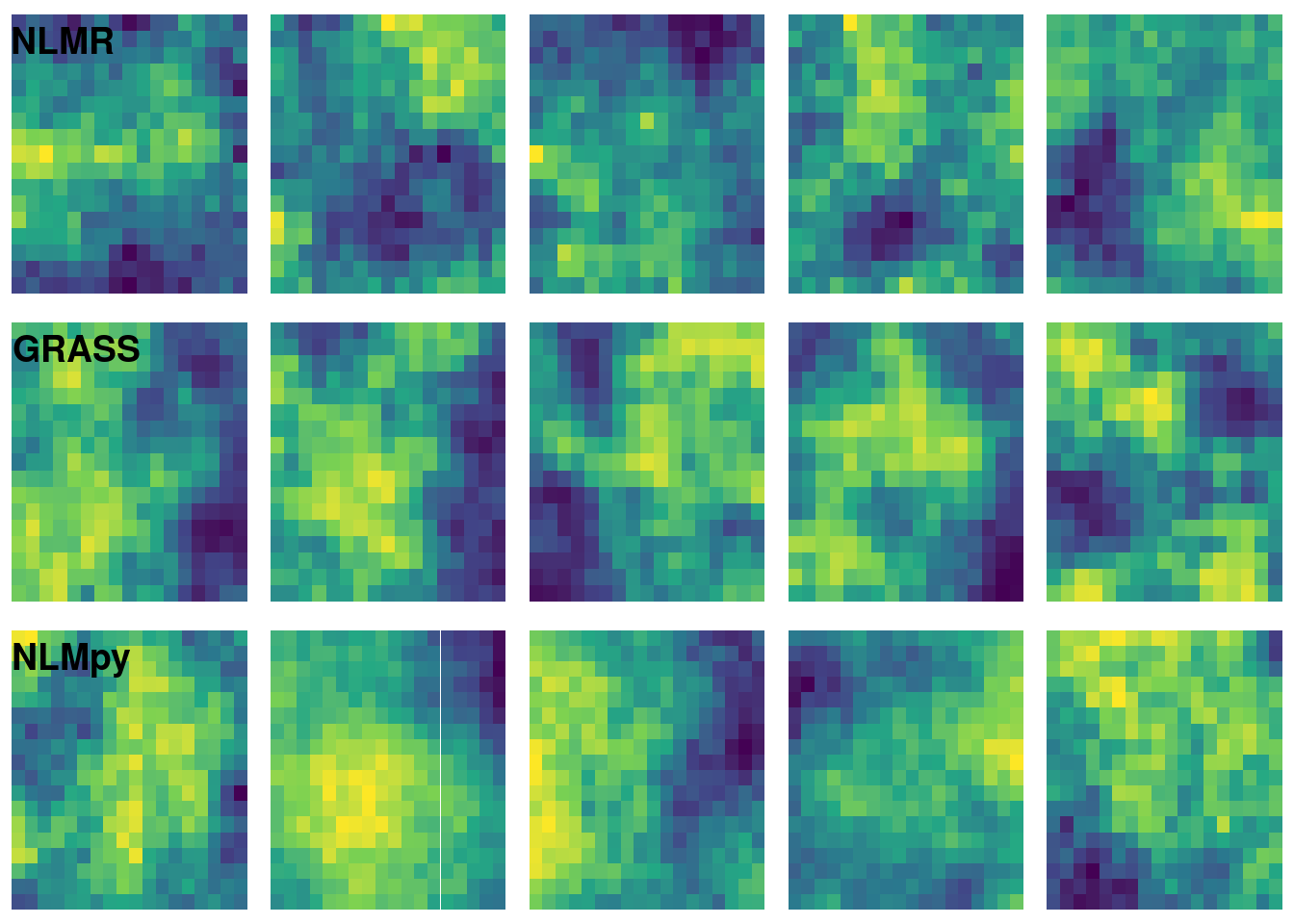# Random Fractal Maps: Software Comparison

Here I show how to use three different software packages to produce neutral landscape models via midpoint displacement. First, I show use of the newly released NLMR package which runs natively in R. Next, I show use of GRASS (via r.surf.fractal) and nlmpy using their respective R wrappers.

library(NLMR)
library(raster)
library(boot)
library(rgrass7)
use_sp()
library(reticulate)
library(ggplot2)
library(purrr)
library(dplyr)
library(cowplot)

## NLMR package1

where the roughness value controls the “level of spatial autocorrelation”.

nlmr_mpd <- function(ncol = 17, nrow = 17, roughness = 0.6) {
nlm_mpd(ncol = ncol, nrow = nrow, roughness = roughness)
}

## GRASS r.surf.fractal2

where the dimension value controls the “fractal dimension of surface (2 < D < 3)”.

initGRASS("/usr/lib/grass78", home = tempdir(), override = TRUE)

raster_template <- as(nlmr_mpd(), "SpatialGridDataFrame")
## Warning in nlm_mpd(ncol = ncol, nrow = nrow, roughness = roughness): nlm_mpd
## changes the dimensions of the RasterLayer if even ncols/nrows are choosen.
grass_mpd <- function(dimension = 2.05){
writeRAST(raster_template, "grass_mpd", flags = "overwrite")
execGRASS("g.region", raster = "grass_mpd")
execGRASS("r.surf.fractal", output = "grass_mpd", flags = "overwrite",
dimension = dimension)
res <- raster::scale(res)
res[] <- boot::inv.logit(res[])
res
}

## NLMpy3

where the h value controls the “level of spatial autocorrelation in element values”.

nlmpy <- import("nlmpy")
nlmpy_mpd <- function(nRow = 18L, nCol = 18L, h = 0.6){
res <- nlmpy$nlmpy$mpd(nRow = nRow, nCol = nCol, h = h)
raster(res, ymx = nrow(res), xmx = ncol(res))
}

## Visual Comparison

The following code chunk generates five neutral landscape simulations using each software option.

# NLMR
nlmr_mpd_res <- lapply(1:5, function(x)
as.data.frame(as(nlmr_mpd(), "SpatialPixelsDataFrame")))
## Warning in nlm_mpd(ncol = ncol, nrow = nrow, roughness = roughness): nlm_mpd
## changes the dimensions of the RasterLayer if even ncols/nrows are choosen.

## Warning in nlm_mpd(ncol = ncol, nrow = nrow, roughness = roughness): nlm_mpd
## changes the dimensions of the RasterLayer if even ncols/nrows are choosen.

## Warning in nlm_mpd(ncol = ncol, nrow = nrow, roughness = roughness): nlm_mpd
## changes the dimensions of the RasterLayer if even ncols/nrows are choosen.

## Warning in nlm_mpd(ncol = ncol, nrow = nrow, roughness = roughness): nlm_mpd
## changes the dimensions of the RasterLayer if even ncols/nrows are choosen.

## Warning in nlm_mpd(ncol = ncol, nrow = nrow, roughness = roughness): nlm_mpd
## changes the dimensions of the RasterLayer if even ncols/nrows are choosen.
names(nlmr_mpd_res) <- 1:5
nlmr_mpd_res <- map2_df(nlmr_mpd_res, names(nlmr_mpd_res), ~ mutate(.x, ID = .y))

# GRASS
grass_mpd_res <- lapply(1:5, function(x)
as.data.frame(as(grass_mpd(dimension = 2.5),
"SpatialPixelsDataFrame")))
names(grass_mpd_res) <- 1:5
grass_mpd_res <- map2_df(grass_mpd_res, names(grass_mpd_res), ~ mutate(.x, ID = .y))

# NLMpy
nlmpy_mpd_res <- lapply(1:5, function(x)
as.data.frame(as(nlmpy_mpd(h = 0.8), "SpatialPixelsDataFrame")))
names(nlmpy_mpd_res) <- 1:5
nlmpy_mpd_res <- map2_df(nlmpy_mpd_res, names(nlmpy_mpd_res), ~ mutate(.x, ID = .y))

# Viz
plot_grid(
ggplot() +
geom_tile(data = nlmr_mpd_res, aes(x = x, y = y, fill = layer)) +
scale_fill_viridis_c() +
facet_wrap(~ID, nrow = 1) + theme_nothing(),
ggplot() +
geom_tile(data = grass_mpd_res, aes(x = x, y = y, fill = grass_mpd)) +
scale_fill_viridis_c() +
facet_wrap(~ID, nrow = 1) + theme_nothing(),
ggplot() +
geom_tile(data = nlmpy_mpd_res, aes(x = x, y = y, fill = layer)) +
scale_fill_viridis_c() +
facet_wrap(~ID, nrow = 1) + theme_nothing(),
nrow = 3, ncol = 1, labels = c("NLMR", "GRASS", "NLMpy"), hjust = -0.1, vjust = 2)I played with the dimension argument of grass_mpd() and the h argument of nlmpy_mpd() to try to get a result that was qualitatively similar to the results of nlmr_mpd(). Note that I have set the size of the nlmpy raster slightly larger than the other rasters. Setting it to 17L causes a strange error that I am hesitant to dig into.

Each of the three options analyzed here have a different specification for controlling surface roughness. This makes analytical comparisons difficult. NLMR definitely is the easiest to use but I suspect that its native R implementation would make it much slower than GRASS for large rasters. NLMpy is also easy to use but the errors I encountered when running a 17 x 17 matrix makes me hesitant to recommend it.